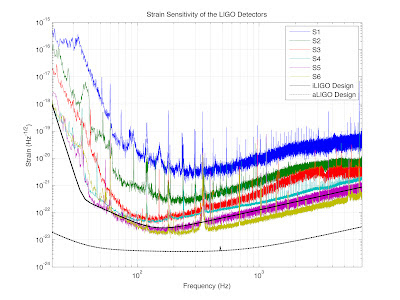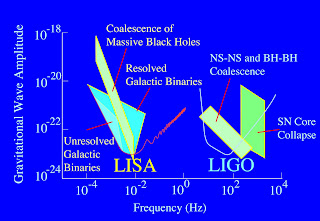## Thursday, May 10, 2012

### Q: Why Isn't LIGO Sensitive to GW From the Sun, Moon, or Planets?

Another question I am often asked at LIGO is why we are looking for gravitational waves from the most violent, energetic events in the Universe when the Sun, Moon, and planets are moving around the Earth right beside us.  The reason for this is that there is simply not enough mass (even in our Sun) moving fast enough to produce detectable gravitational waves.  The key here is "fast enough".  Let's look at the sensitivity of LIGO to figure out what is "fast enough"...Click on this plot for a larger image.

These are the sensitivity curves for all of the LIGO science runs (labeled S1, S2, etc. in chronological order), the design sensitivity for Initial LIGO (iLIGO), and Advanced LIGO (aLIGO).  Remember, like in golf, a lower number is better (more sensitive).  There is a little more to reading this graph:
• The vertical (Y) axis is in units of strain.  That is just the change in length of LIGO's arms divided by their original length (4 km or 4000 m).  The numbers on this axis on in scientific notation (10-18, etc.).  The negative in front of the super-scripted number (exponent) means the number of places after the decimal place.  For example, 10-3 is the same as 0.001.  103 (note there isn't a negative sign here so this is the number of zeros before the decimal place) is 1000.  10-21 is a strain that corresponds to a change in length of the arms of 1000x smaller than the diameter of a proton.
• The horizontal (X) axis is frequency which is measured in units of Hertz (Hz, how many crests of a wave pass by every second).  This is also in scientific notation (like mentioned above).  The spacings of the lines are uneven because this is in log (logarithmic) scale.  The line labeled 102 is 100 Hz, the next unlabeled line is 200 Hz, the next unlabeled is 300 Hz, etc.  Each labeled line is 10x more than the labeled line before it.
The frequencies where LIGO is most sensitive is between 100-1000 Hz (102 - 103 Hz).  At lower frequencies (to the left on the plot) the sensitivity curve takes a sharp turn up to lower sensitivities (remember, a higher plot means lower sensitivity).  This is due to the seismic vibrations that are constantly present on the Earth mostly from the microseism.  The only way to eliminate this noise is to place a gravitational-wave detector in space.

You can see from the sensitivity plot that for our last science run (S6, the yellow-green curve) achieved a sensitivity better than the Initial LIGO design (the black curve just above it).  For Advanced LIGO (the lowest black line) there are several configurations that it can be operated in (more details are here), but the curve represented here produces the best sensitivity over a broad frequency range and is likely to be the general operation state.  Advanced LIGO will have even better sensitivity at lower frequencies, but not enough to be sensitive to objects in our Solar System.

So, what frequency would be the gravitational waves from Sun?  The Earth orbits the Sun once every year (365.25 days or 31,556,926 seconds).  This gives a frequency (f=1/year) of 3.1689x10-8 Hz or 0.000000031689 Hz.  This is WAAAAY too low of a frequency for LIGO or for any proposed space based detector (like the recently canceled LISA which only goes down to about 10-4 Hz or 0.0001 Hz):Plot comparing the sensitivity curves of Initial LIGO and LISA.

Therefore, since nothing with great mass moves in our Universal neighborhood with high frequencies, we must look deeper for things like stars exploding an black holes colliding.  These are much more interesting that just observing the gravitational waves from the Solar System; they really wouldn't tell us much new information about the Universe.  At LIGO we are not just seeking to make the first direct detections of gravitational waves, but we are trying to use these gravitational waves as a new way to do astronomy!